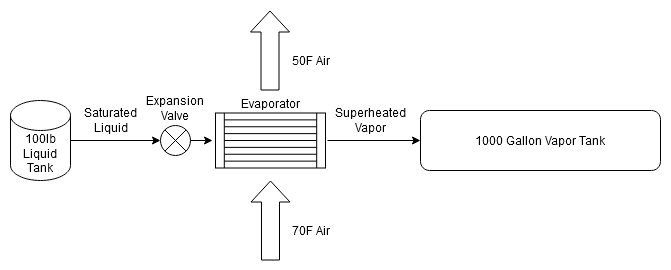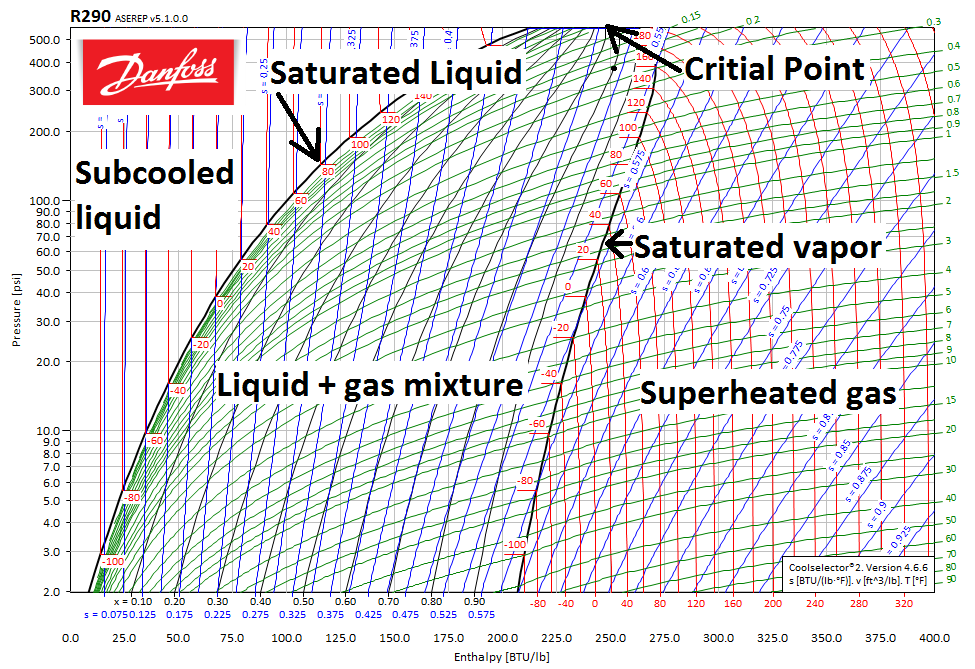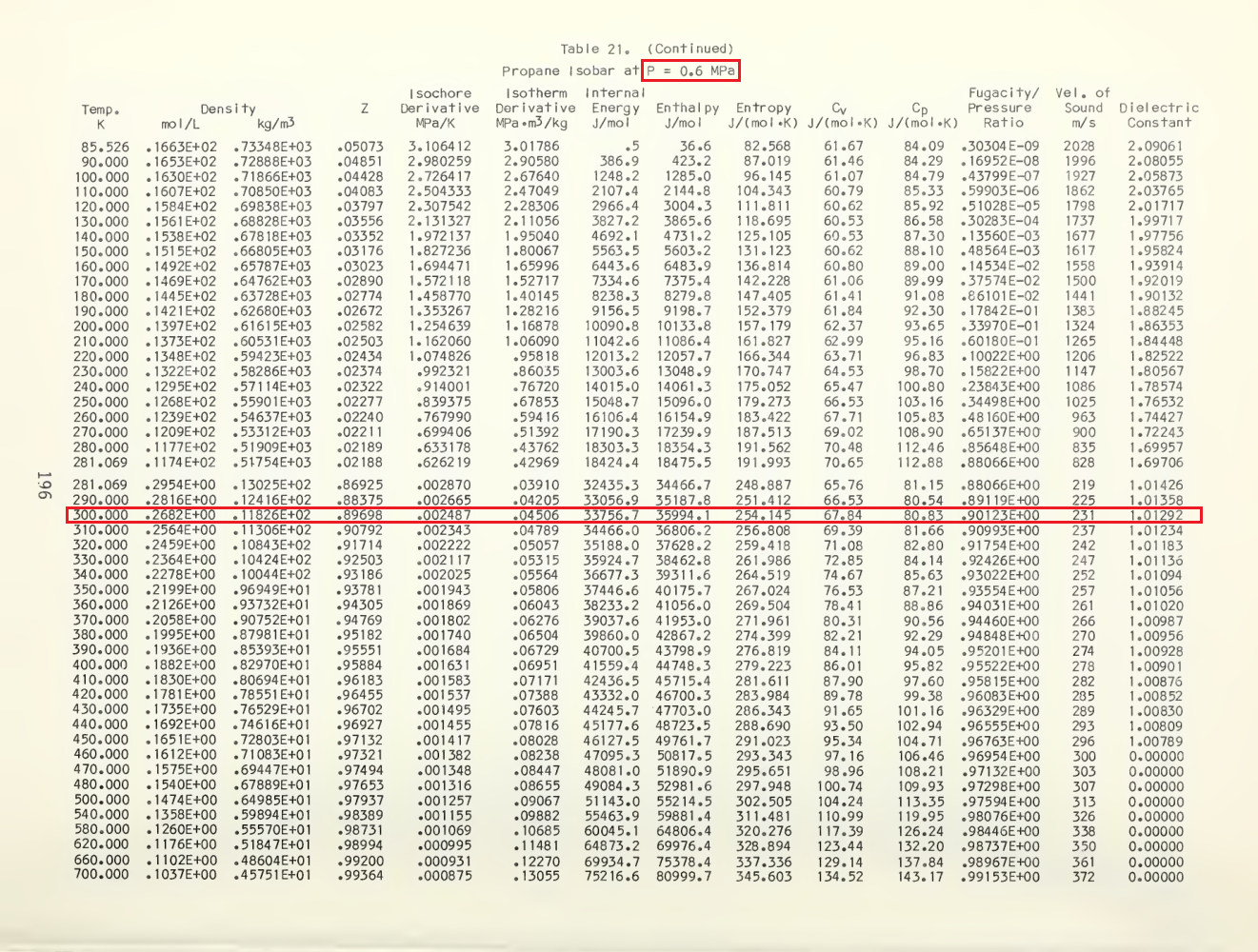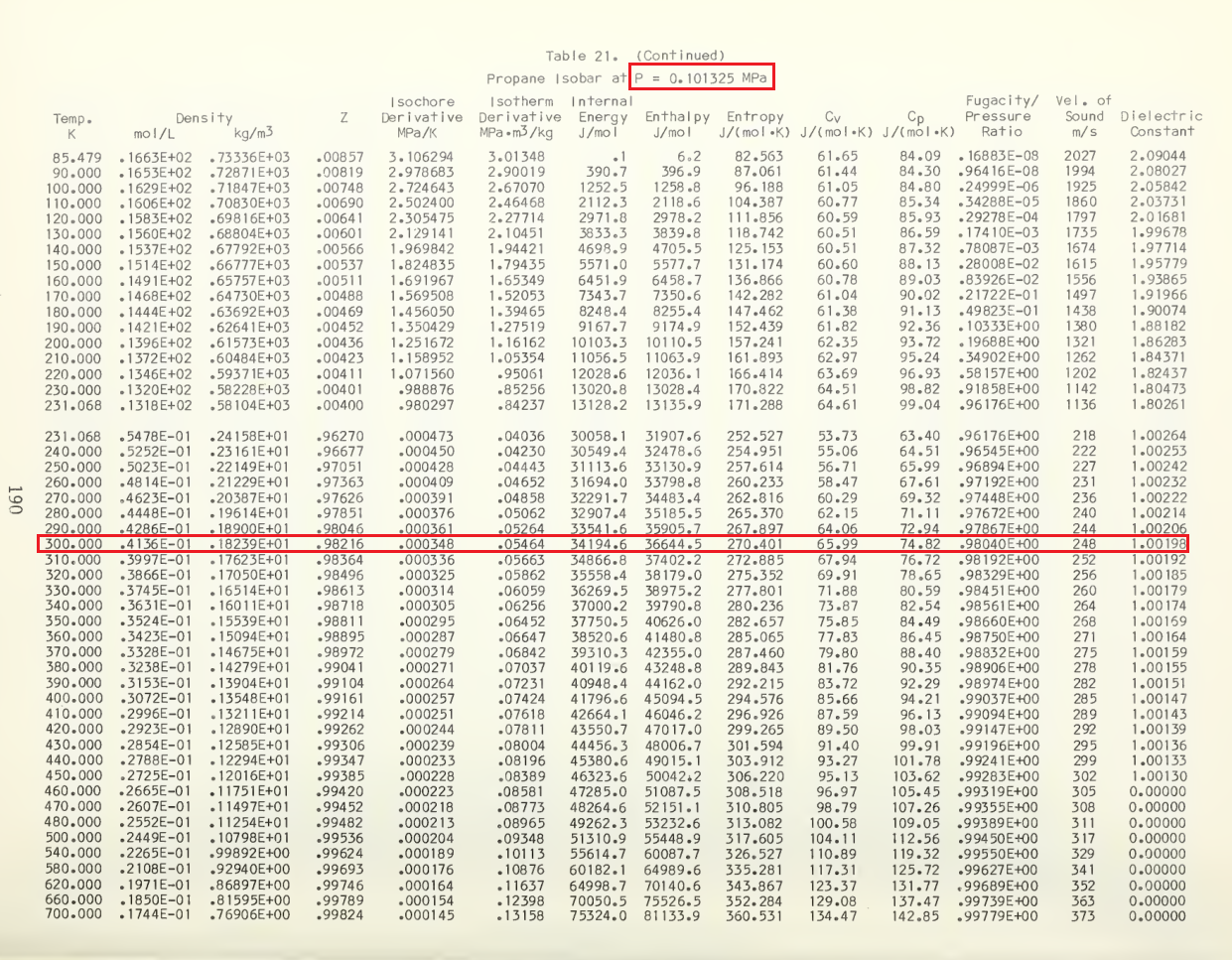# Bad Idea: Propane Thermal Storage

### 2022

If you have an off-grid cabin in a hot and humid climate that you want air conditioning, you'll need a large solar and, more importantly from the perspective of system longevity, battery setup to run it overnight. Vapor-compression refrigeration systems provide cooling by taking a high pressure saturated liquid, passing it through an expansion valve to the low pressure side of the system, and evaporating the liquid into a gas. Normally this gas is then compressed and subsequently cooled by passing it through a condenser to generate the high pressure saturated liquid that we started with, but you can continue to evaporate liquid refrigerant as long as the pressure of the low side of the system is lower than the pressure of the high side of the system. To achieve this, you need a large pressure vessel to contain the evaporated gas on the low pressure side.

Propane is actually a pretty good refrigerant gas and is called R-290 in this context. Propane can in some cases be used to replace more common refrigerants like R-134a, and it has a significantly lower global warming potential (~9.5 vs. 1300). It is also comparatively cheap and unregulated, and large used tanks (the limiting factor for this idea) can be found somewhat inexpensively which is why I considered it for this thought experiment.Diagram of System in 'Discharge' Mode

Not shown in the above diagram is the compressor and condenser that would be operated during the day when there was sufficient solar power to refill the liquid tank and pump down the vapor tank.

### Overview of Why This is a Bad Idea:

• You can store only around 12,000 BTU of cooling worth of propane gas in a 1000 gallon tank.
• This is equivalent to a small window AC running for two hours or a large window AC running for one hour.
• Going by the 20 BTU/h per square foot, this would be sized to cool only 600 square feet for an hour.
• Even used, a 1000 gallon propane tank will cost ~\$1000.
• A \$75 275 gallon tank of water can absorb 40,000 BTU (3x as much) heat just from heat capacity between 0.1C and 10C.
• Compressor oil would need to be fully separated from the gas going into the condenser to prevent accumulation in the liquid tank.
• Efficiency will be lower because more work needs to be done to bring the vapor tank down to ambient pressure vs. normal low side pressure of a continuously operating system.
• When the system begins to operate with the vapor tank its lowest pressure, evaporator temperature will be -45F which may result in icing.
• Propane is flammable.

## Calculations

Two things need to be known to calculate the thermal capacity of a system like this: How much propane gas can be stored at a given pressure and how much heat can be absorbed to evaporating that quantity of propane. Because the start and end pressures are not at ambient and because additional heat is absorbed by the superheated gas, you can't just use the latent heat of evaporation to calculate this. The latent heat of evaporation at the boiling point of propane at ambient is 184 BTU/lb, in contrast, the actual heat that can be absorbed with this setup (calculated later on) is ~141 BTU/lb. Since propane is not an ideal gas, you need to either use an equation of state or look at a table to find its actual density at a given pressure. The compressibility of propane is at worst 0.9 over this pressure and temperature range, so ideal gas assumptions would introduce ~10% error in the calculation.

### Enthalpy Change

Assumptions:

• The temperature of the liquid tank is 80F. It will absorb heat from the environment to maintain this temperature.
• The temperature of the vapor leaving the evaporator is 50F.
• The system is only effective with evaporator temperatures under 50F (a typical AC unit will have a <45F evaporator).
• The vapor tank is in an 80F environment.
• Vapor enters the vapor tank at 50F and warms up to 80F as the tank absorbs heat from the environment.

Pressure-enthalpy diagrams are commonly used to calculate refrigeration cycles. I generated a PH diagram using a free software from Danfoss called Coolselector-2. This diagram shows how enthalpy changes as pressure and temperature change. Specifically for this application, it shows the enthalpy change between a saturated liquid at 80F (the liquid tank) and a superheated vapor at 50F.Pressure Enthalpy Diagram Overview

The enthalpy of the vapor at 50F varies with final pressure. At the start with the vapor tank at 14 PSI (atmospheric pressure), the final enthalpy is 260 BTU/lb. At the end at 88 PSI, the enthalpy is 252 BTU/lb. Since this is a small range, I assumed an average number of 256 BTU/lb for my calculations. The starting enthalpy is 115 BTU/lb, so the average heat absorbed per pound is 256 - 115 = 141 BTU/lb. Here is an illustration of the throttling, evaporation, and superheating process as the vapor tank fills that I made with Python:Enthalpy Change as Vapor Tank Fills

The starting point does not change as the vapor tank fills because the liquid tank is at constant temperature. The blue arrow is the expansion process. As the saturated liquid expands through an expansion valve, it turns into a mixture of liquid and vapor at a lower temperature and pressure. This is treated as an adiabatic process (no heat is exchanged in the expansion valve), so enthalpy does not change. The red line represents heat being absorbed by the refrigerant as it is warmed in the evaporator. After the liquid has fully evaporated, the still cold vapor is superheated to 50F before leaving the evaporator (the evaporator is the heat exchanger cooling the conditioned air.)

As the vapor tank fills, its pressure goes up until a stopping point of 88 PSI. This is the pressure at which propane will boil at 50F. Since I assumed that the system is only effective with evaporator temperatures less than 50F, this is where my calculation stops. The fundamental limit on maximum evaporator temperature is the desired air temperature of the conditioned space. If you want to cool a room to 70F, the evaporator must be less than 70F. To actually transfer heat with reasonable airflow, there must be a temperature delta between the evaporator and the incoming air which is why I chose 50F.

### Storage Capacity

By the ideal gas law, the density of propane gas at 88 PSI and 80F will be 10.72 kg/m^3, however, propane is not an ideal gas and empirically developed tables show that the density at 80F and 87 PSI is 11.83 kg/m^3 which interpolates to 11.96 kg/m^3 at 88 PSI. At this density, a 1000 gallon tank can hold 99.9 lbs of propane gas.Propane Properties at 0.6 MPa from "NBS Monograph 170"

Since its a good idea to keep all parts of the system at or above atmospheric pressure, the minimum pressure of the vapor tank is 14 PSI meaning that there is some quantity of propane in it at the beginning. The density of propane at 80F and ambient pressure is 1.824 kg/m^3 meaning that a 1000 gallon tank starts with ~15 lb of propane vapor. Note that at lower pressures, the compressibility of the gas is much closer to 1 meaning that it behaves more closely to an ideal gas.Propane Properties at Atmospheric Pressure from "NBS Monograph 170"

The amount of propane that can be stored in a 1000 gallon tank is therefore around 85 lbs. Multiplying that mass by 114 BTU/lb as calculated in the previous section comes out to 12.0 kBtu.

## Comparison to Water

12 kBTU per 1000 gallons of storage is a really crappy number compared to using just the heat capacity of water with no phase changes. 1000 gallons of 34F water will absorb 135kBtu when heated to 50F. This means that 100 gallons of water has more heat storage potential for refrigeration (13.5 kBtu) than 1000 gallons of propane gas. If 1/3 of that water were frozen, the heat capacity increases to 53.6 kBTU which is starting to get into the useful territory for an overnight thermal storage system. You can buy 275 gallon IBC totes on Craigslist for \$75. Even if only 10% of the volume could be utilized for ice, the storage capacity is 70.25 kBtu between 0C and 10C.

An additional benefit of this storage is that the compressor only needs to operate between 40 and 140 PSI (assuming propane as the refrigerant still) while the compressor needs to operate between 14 and 140 PSI when pumping the vapor storage tank all the way down to atmospheric pressure.